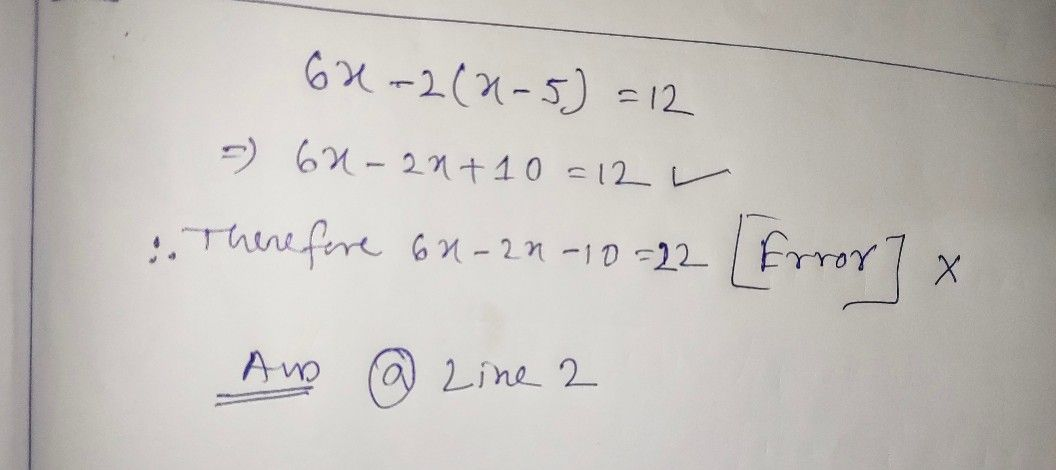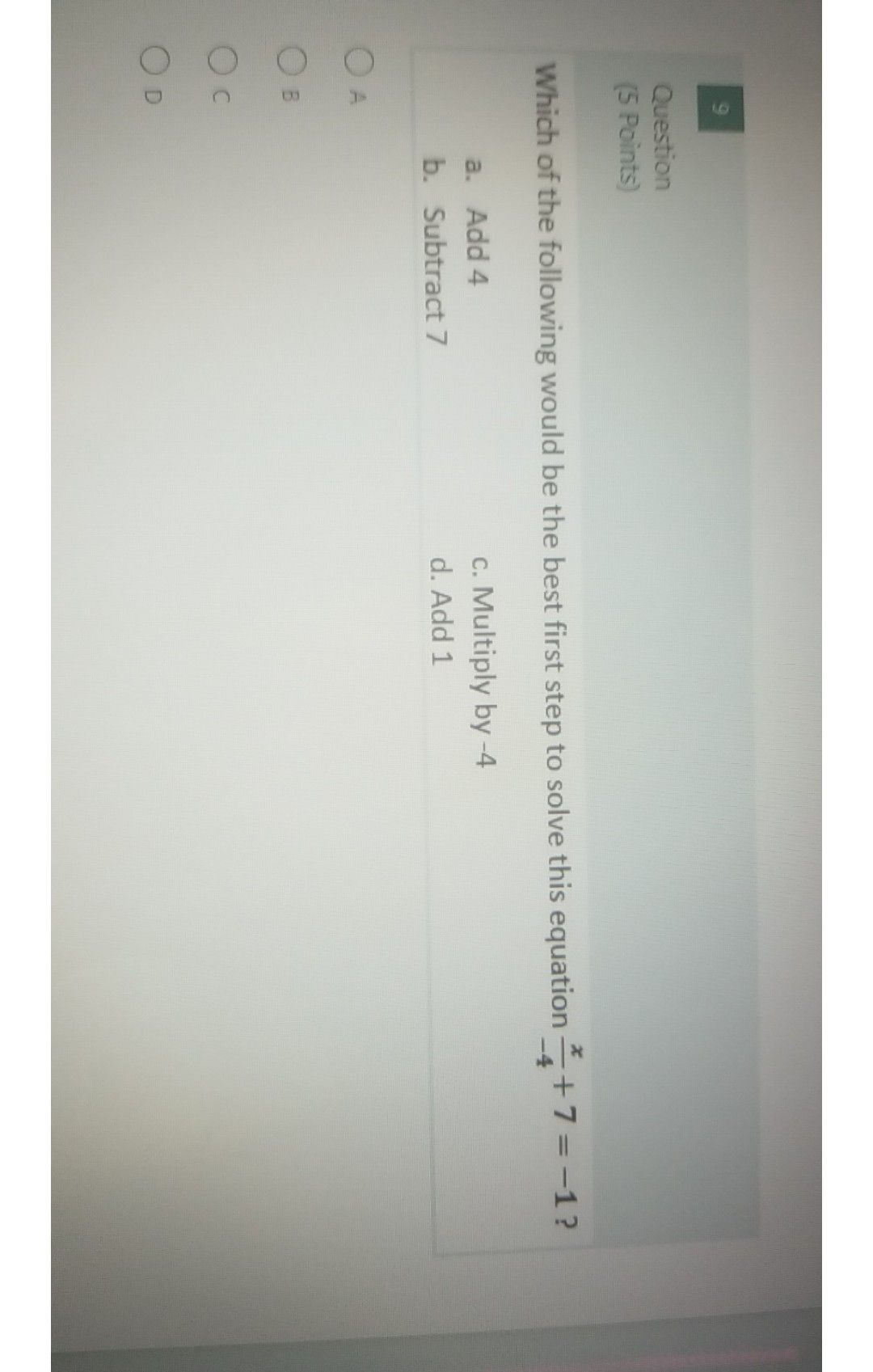Symbol
ProblemQuestion $\left(5$ Points) In the problem below, which line contains an error? a. Line $2$ Line $1:$ $6x-2\left(x-5\right)=12$ b. Line 3 Line $2$ $6x-2x-10=22$ Line $3$ $4x-10=22$ C. Line $4$ Line $4$ $4x=32$ d. Line 5 Line $5$ $x=8$ $\square$ $-$ A O B $C$ $○$ $\square$
Algebra
Question content